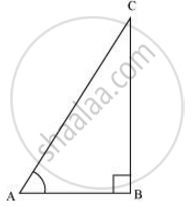Share
Notifications

View all notifications
Books Shortlist
Your shortlist is empty

# State whether the following are true or false. Justify your answer. The value of tan A is always less than 1. - CBSE Class 10 - Mathematics

Login
Create free account

Forgot password?

#### Question

State whether the following are true or false. Justify your answer.

The value of tan A is always less than 1.

#### Solution

Consider a ΔABC, right-angled at B.tan A = ("Side opposite to"angle A)/("Side adjacent to"angleA)

= 12/5

But 12/5 > 1

∴tan A > 1

So, tan A < 1 is not always true.

Hence, the given statement is false.

Is there an error in this question or solution?

#### APPEARS IN

NCERT Solution for Mathematics Textbook for Class 10 (2019 to Current)
Chapter 8: Introduction to Trigonometry
Ex.8.10 | Q: 11.1 | Page no. 181
Solution State whether the following are true or false. Justify your answer. The value of tan A is always less than 1. Concept: Trigonometric Ratios.
S